# （算法）通俗易懂的字符串匹配KMP算法及求next值算法

1、最基本的朴素算法
2、优化的KMP算法
3、应算法需要定义的next值
4、手动写出较短串的next值的方法
5、最难理解的、足足有5行的代码的求next值的算法

### 二、朴素算法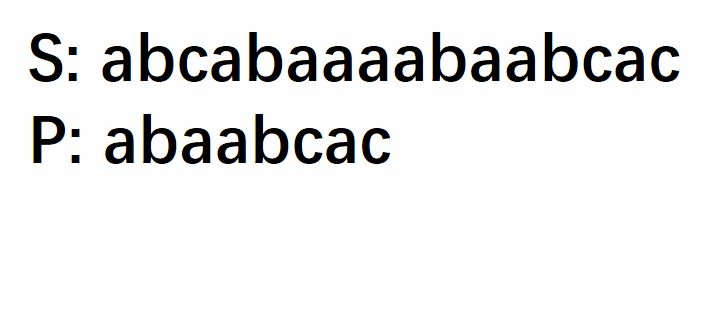#include<stdio.h>
int Index_1(char s[],int sLen,char p[],int pLen){//s为主串，sLen为主串元素个数，p为模式串，pLen为模式串的个数
if(sLen<pLen)return 0;
int i = 1,j = 1;
while(i<=sLen && j<=pLen){
if(s[i]==p[j]){i++;j++;}
else{
i = i-j+2;
j = 1;
}
}
if(j>pLen) return i-pLen;
return 0;
}
void main(){
char s[]={' ','a','b','c','a','b','a','a','a','a','b','a','a','b','c','a','c'};//从序号1开始存
char p[]={' ','a','b','a','a','b','c','a','c'};
int sLen = sizeof(s)/sizeof(char)-1;
int pLen = sizeof(p)/sizeof(char)-1;
printf("%d",Index_1(s,sLen,p,pLen));
}


### 三、改进的算法——KMP算法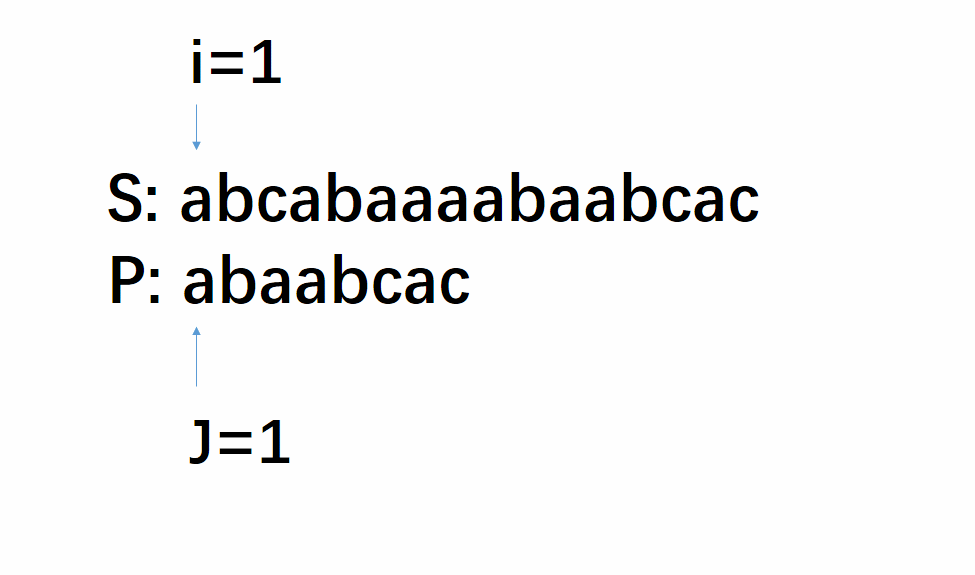KMP算法： 每次失配，S串的索引i不动，P串的索引j定位到某个数。T(n)=O(n+m)，时间效率明显提高

• 比如，Pj处失配，绿色的是Pj，则我们可以确定P1…Pj-1是与Si…Si+j-2相对应的位置一一相等的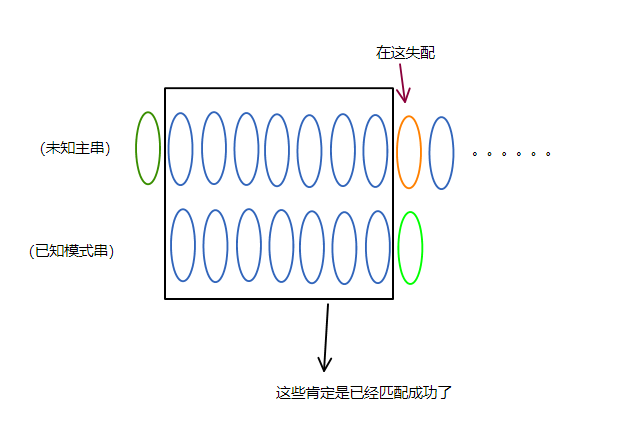• 假设P1…Pj-1中，P1…Pk-1与Pj-k+1…Pj-1是一一相等的，为了下面说的清楚，我们把这种关系叫做“首尾重合”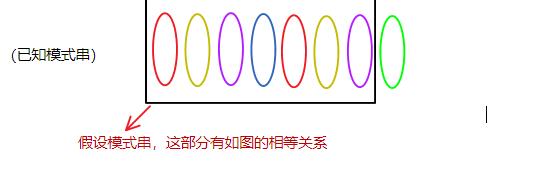• 那么可以推出，P1…Pk-1与Si…Si+j-2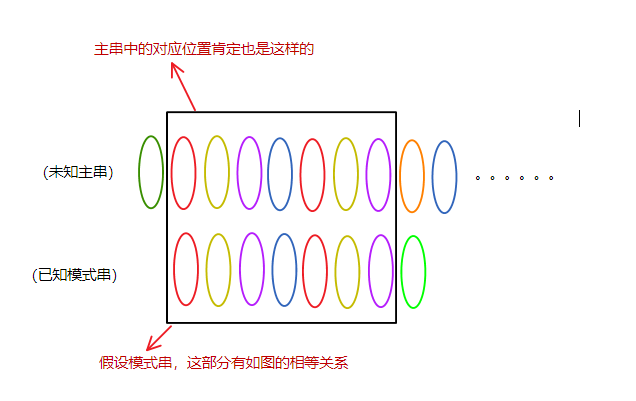• 显然，接下来要做的就是把模式串右移了，移到哪里就不用多说了：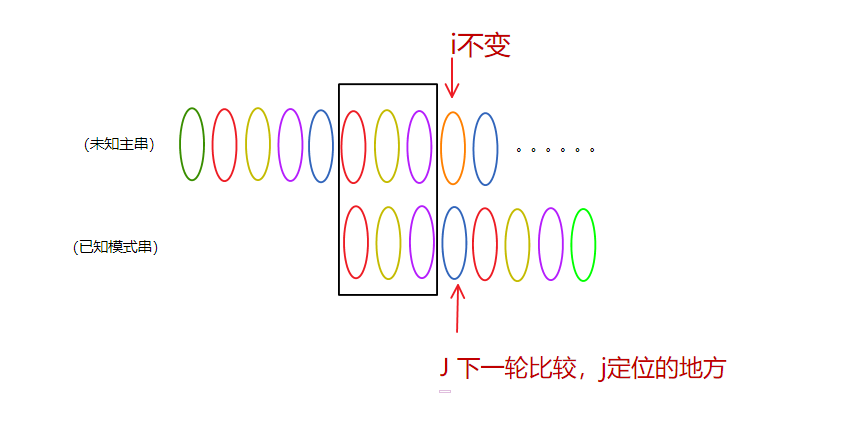• 为了表示下一轮比较j定位的地方，我们将其定义为next[j]，next[j]就是第j个元素前j-1个元素首尾重合部分个数加一，当然，为了能遍历完整，首尾重合部分的元素个数应取到最多，即next[j]应取尽量大的值，原因挺好理解的，可以想个例子模拟一下，会完美跳过正确结果。在上图中就是绿色元素的next值为蓝色元素的序号。也即，对于字符串P，next=4。如此，再看一下上面的动图是不是清楚了不少。

• 最后，如果我们知道了一个字符串的next值，那么KMP算法也就很好懂了。相比朴素算法，当发生失配时，i不变，j=next[j]就好啦！接下来就是怎么确定next值了。

### 四、手动写出一个串的next值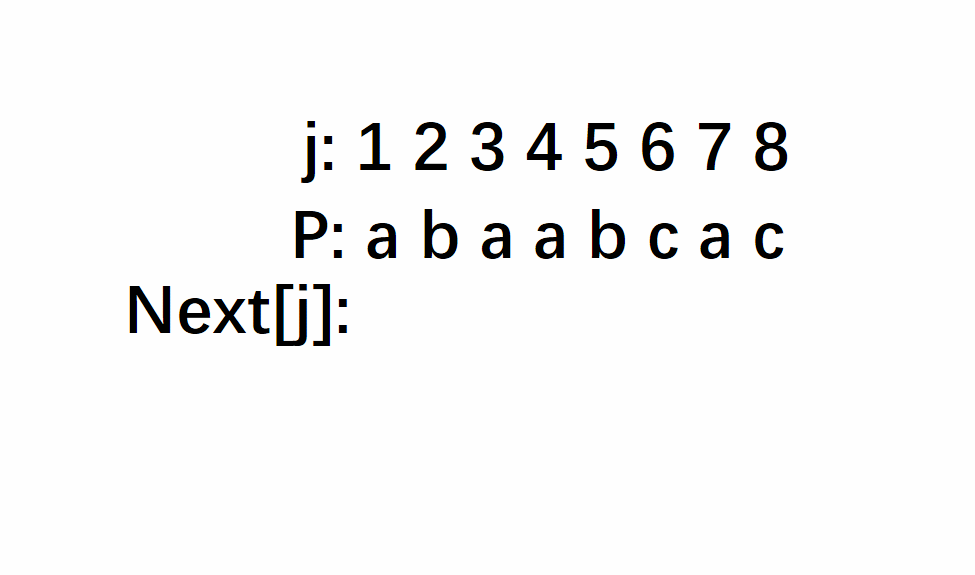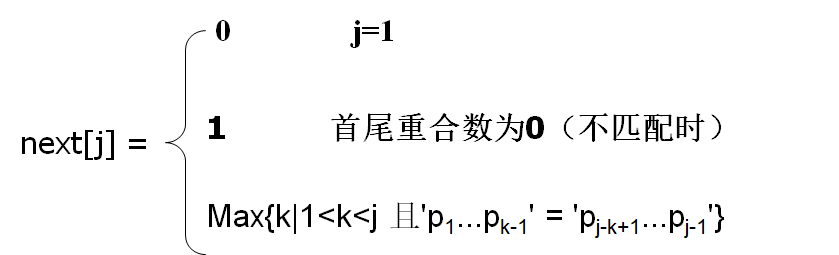### 五、求next的算法

int GetNext(char ch[],int cLen,int next[]){//cLen为串ch的长度
next = 0;
int i = 1,j = 0;
while(i<=cLen){
if(j==0||ch[i]==ch[j]) next[++i] = ++j;
else j = next[j];
}
}

• 还是先由一般再推优化：
直接求next[j+1]（至于为什么是j+1，是为了和下面的对应）
根据之前的分析，next[j+1]的值为pj+1的前j个元素的收尾重合的最大个数加一。即需要满足两个条件，把它的值一步步“检验”出来。一是“个数最多”的，因此要从可能的最大值开始验；二是“首尾重合”，因此要一一对应验是否相等。
不难理解，next[j+1]的最大值为j，所有我们从next[j+1]=j开始“验证”。有以下优先判断顺序：
if(P1…Pj-1 == P2…Pj) => next[j+1]=j
else if(P1…Pj-2 == P3…Pj) =>next[j+1]=j-1
else if(P1…Pj-3 == P4…Pj) =>next[j+1]=j-2

else if(P1P2 == Pj-1Pj) => next[j+1]=3
else if(P1 == Pj-1) => next[j+1]=2
else if(P1 != Pj-1) => next[j+1]=1

每次前去尾1个，后掐头1个，直至得到next[j+1]

• 再进一步想，next值是一个“工具”，我们单独的求next[j+1]是完全没有意义的，就是说要求next就要把所有j的next求出来。所有一般的，我们都是已知前j个元素的next值，求next[j+1]，以此递推下去，求完整的next数组
但是，上面的思考过程还是最根本的。所以问题变为两个：知道前j个元素的next的情况下，
①next[j+1]的可能的最大值是多少（即从哪开始验证）
②某一步验证失败后，需要“前去尾几个，后掐头几个？”（即本次验证失败后，再验证哪个值）
看一下的分析：

1、next[j+1]的最大值为next[j]+1。

2、如果Pk1≠Pj，那么next[j+1]可能的次大值为next[next[j]]+1，以此类推即可高效求出next[j+1]

①求next[j+1]，设值为m

⑤第二第三步联合得到:
P1…Pk2-1 = Pk1-k2+1…Pk1-1 = Pj-k1+1…Pk2-k1+j-1 = Pj-k2+1…Pj-1 即四段重合
⑥这时候，再判断如果Pk2=Pj，则P1…Pk2-1P~k2 = Pj-k2+1…Pj-1Pj，则next[j+1]=k2+1；否则再取next[k2]=k3…以此类推

1、要求next[k+1] 其中k+1=17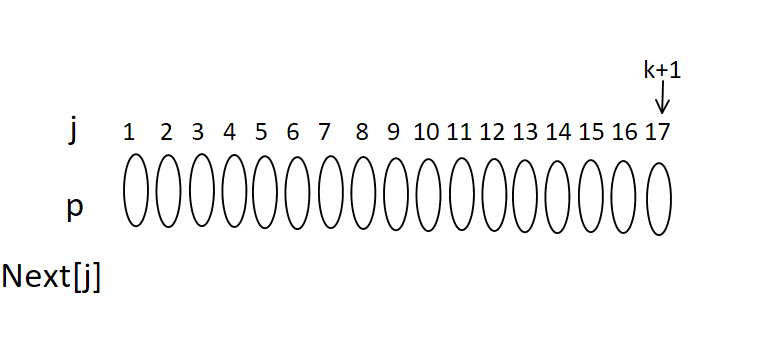2、已知next=8，则元素有以下关系：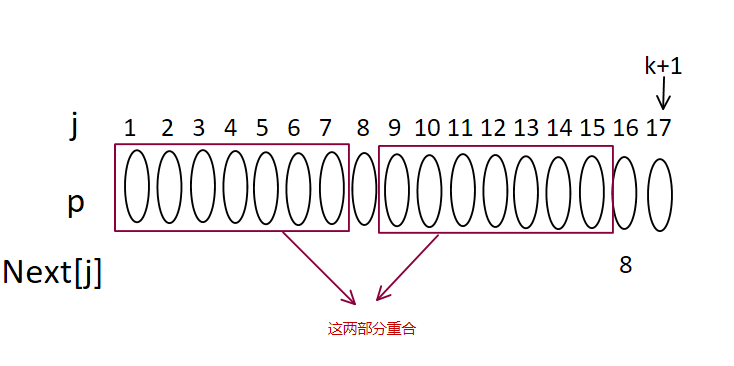3、如果P8=P16，则明显next=8+1=9
4、如果不相等，又若next=4，则有以下关系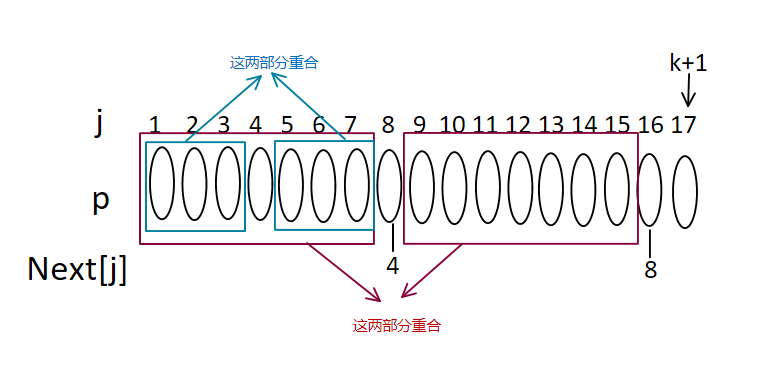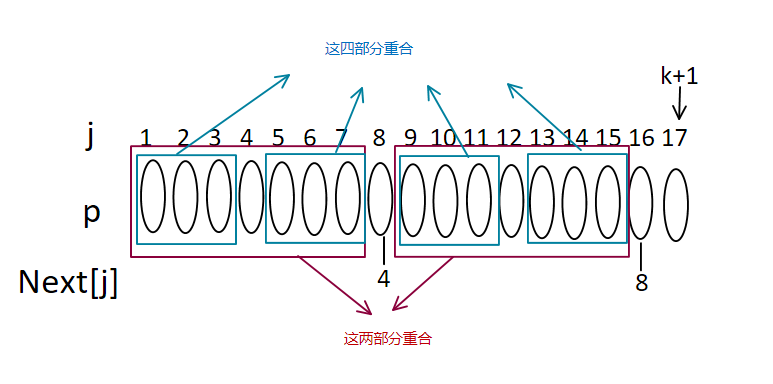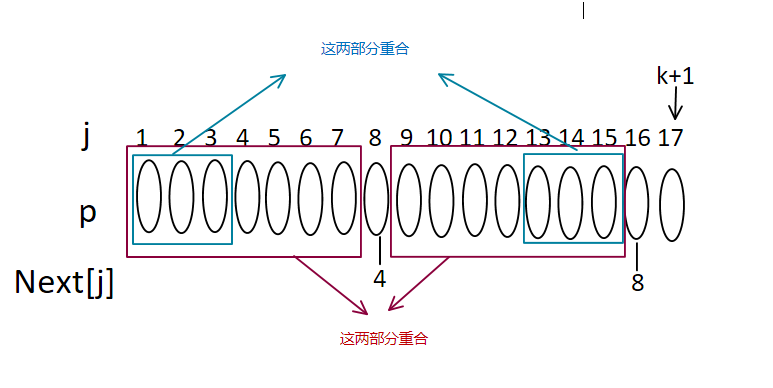5、现在在判断，如果P16=P4则next=4+1=5，否则，在继续递推
6、若next=2，则有以下关系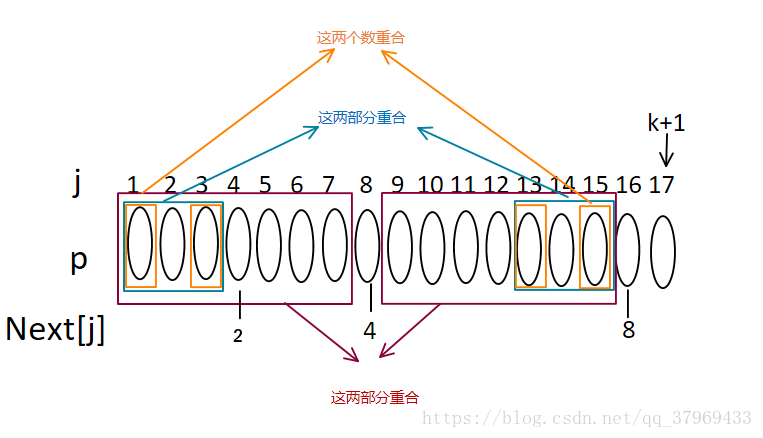7、若P16=P2，则next=2+1=3;否则继续取next=1、next=0；遇到0时还没出结果，则递推结束，此时next=1。最后，再返回看那5行算法，应该很容易明白了！

08-166万+03-2215万+
02-0724万+
09-2423万+
08-306372
04-241656
09-057693
04-101万+